# Summary of Chapter 6, Decision Trees 第六章总结 决策树

What is Decision Tree? How is it different from other models? Let’s understand our first non-linear model, non-parametric model. 何为决策树？跟其他模型区别在哪儿？来了解我们第一个非线性的非参数的模型。

In this chapter summary, I will briefly introduce how Decision Tree in Scikit-Learn works, as well as measurement of impurity. 在这节总结中，我将展示决策树的简单原理。

#### Table of Content 内容提要

• CART 分类和回归树
• Impurity 不纯度
• Cost function 代价函数
• Probability 概率
• Regularization 正则
• Regression 回归

Like SVMs (introduced in Chapter 5 Summary), Decision Trees are versatile Machine Learning algorithms that can perform both classification and regression tasks. They are very powerful algorithms, capable of fitting complex datasets.

### What is a Decision Tree 决策树是什么

What is a decision tree? Decision Trees are tree-like models, where each node contain a subset of the datasets and, if it is a nonleaf node, makes decisions on how the subset can be further split into more branches.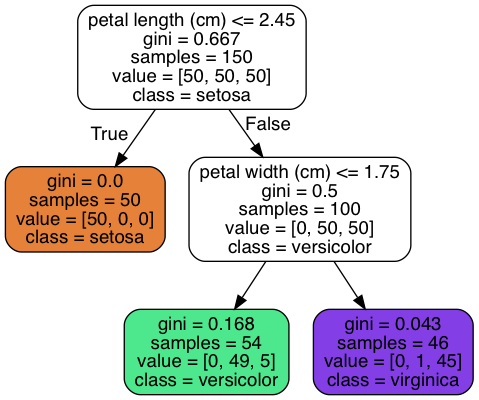In the chart above, there are 150 samples in the top-level node. These samples are split into two subsets based on whether their pental length<=2.45.

### Classification and Regression Tree (CART) 分类和回归树

There are many algorithms for Decision Trees. Scikit-Learn uses the CART algorithm, which produces only binary trees: nonleaf nodes always have two children. As you can tell from the name, CART can be applied to both classification and regression problems. You can probably tell that there are also algorithms produces more than just two children; one example is ID3.

### Impurity 不纯度

At nonleaf nodes, Decision Trees make decision on how to further split the dataset based on certain criteria. How are these criteria decided? Intuitively, in a classification problem, we wish a split could separate instances of different classes as distinct as possible. In the best case, one subset will contain instances from one class, the other subset the other class. In another word, we hope the subset as pure as possible. And the best split should give the least impurity.

Now we need some measurements of impurity. Here are two common ones.

• gini_impurity 基尼不纯度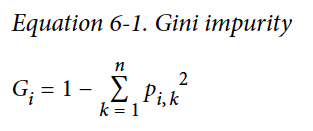• Entropy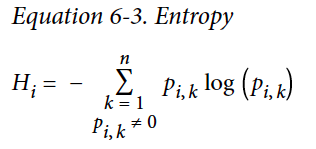subscript $i$ is for nodes, subscript $k$ for classes. For example, in the decision tree shown just now, at the top-level node, $p_{i,1}=p_{i,2}=p_{i,3}=\frac{50}{150}=0.33$, $G_i = 1-\sum_{k=1}^3p_{i,k}^2=1-3\cdot 0.33\cdot 0.33=0.667$.

It is easy to tell that these two metric changes almost the same way when $p_{i,k}$ changes. So they are almost equivalent.

A reduction of entropy is often called an information gain.

### Cost function for Classification 分类问题的代价函数

To minimize the impurity, we can subsequently define the cost function of each split as 以着最小化不纯度的标准，定义代价函数如下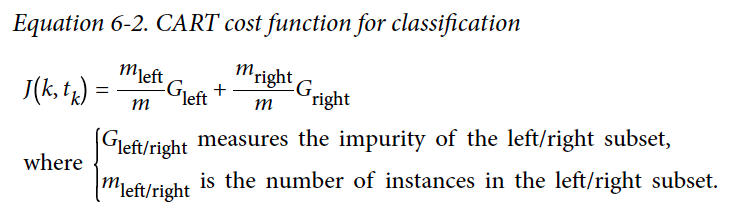### Regularization 正则

Now we know how to split at a single node. Iterating this process on all the produced children node generates a decision tree.

When do we stop splitting? If we only stop when there is no more impurity, we are obviously just remembering the mapping, which is an extreme case of overfitting. To regularize, there are various hyperparameters to put an early stop to the decision tree growing. Common hyperparameters are max_depth, min_sample_split, etc. And again, cross-validation is our good friend on checking overfitting.

### Probability 概率

A Decision Tree can also estimate the probability that an instance belongs to a particular class k: first it traverses the tree to find the leaf node for this instance, and then it returns the ratio of training instances of class k in this node.

### Regression 回归

We have previously mentioned that CART can also be used for regression problem. The CART algorithm works mostly the same way as earlier, except that instead of trying to split the training set in a way that minimizes impurity, it now tries to split the training set in a way that minimizes the MSE.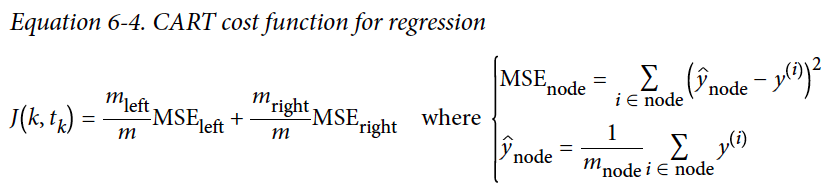#### Discussion 讨论

A single decision tree model suffers a few issues. For example, the split can only be orthogonal to the axes, which makes it suffer from dataset rotation. Besides, Decision Trees are sensitive to small variations in the training data.
How to overcome these issues? You will see it in the next summary, the Ensemble methods. 单一的决策树面临一些问题。比如，分割一定是垂直于坐标轴的，数据集相对于坐标轴的旋转会导致些困扰。决策树也对训练集的改变很敏感。如何解决这些问题呢？敬请期待下篇总结：集成方法。

This article is part of a series of summaries on the book Hands-On Machine Learning with Scikit-Learn and TensorFlow. The summaries are meant to explain machine learning concepts and ideas, instead of covering the maths behind the models.

Written on May 18, 2018